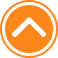×

PlaySubtraction Math Challenge70%Device: 💻 Pc   📱 Mobile
Rating: 70%
🎲Subtraction Math Challenge

Subtraction Math Challenge is fun math game suitable for all ages. I hope you like math and this game is just to test your math skills. Ten seconds to answer each quastion. Have fun playing. Subtraction Math Challenge is a great game that you can play for free at in friv50000.net. Regardless Subtraction Math Challenge game, we provide many cool Friv 50000 games to play for free.

🎮 Instructions

Use Left Mouse button to Play

📂 Categories

puzzle, arcade, mathka, numbers, school, thinking, mathematical, mathematics, igrice, mathematic, math..

👌 Recommended
📌 Tags